# Application of derivatives Questions and Answers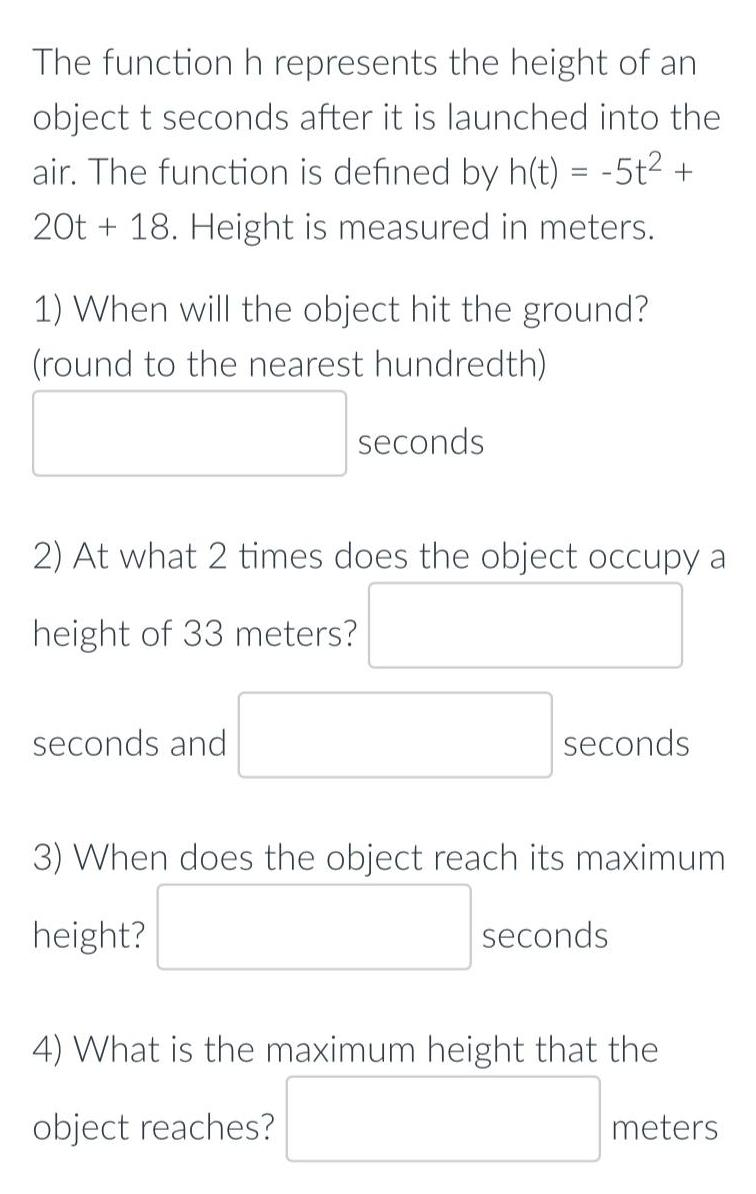Math
Application of derivatives
The function h represents the height of an object t seconds after it is launched into the air. The function is defined by h(t) = -5t² + 20t+18. Height is measured in meters. 1) When will the object hit the ground? (round to the nearest hundredth) seconds 2) At what 2 times does the object occupy a height of 33 meters? seconds and seconds 3) When does the object reach its maximum height? seconds 4) What is the maximum height that the object reaches? meters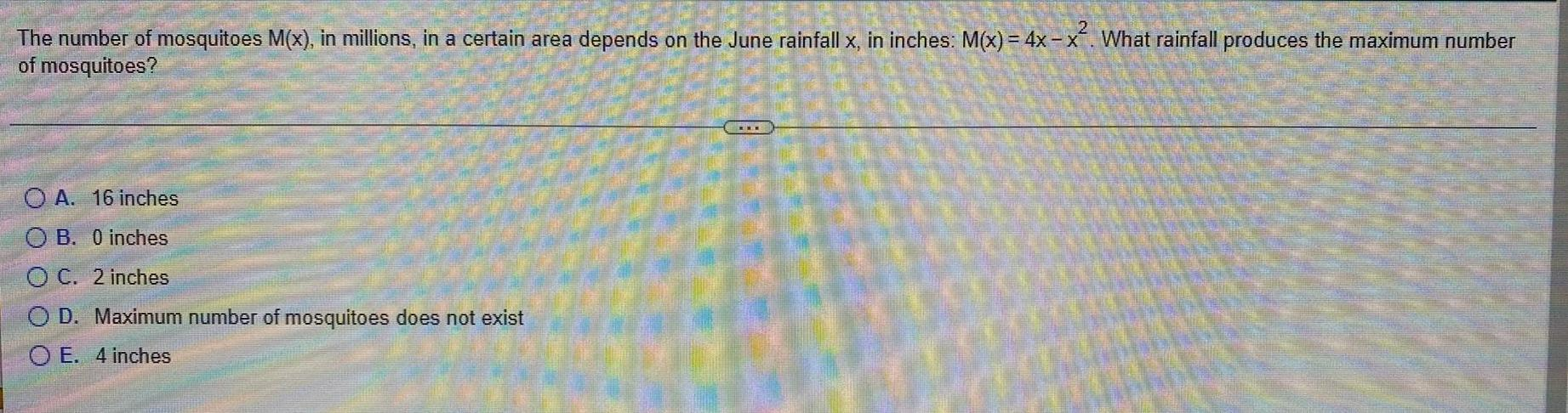Math
Application of derivatives
The number of mosquitoes M(x), in millions, in a certain area depends on the June rainfall x, in inches: M(x) = 4x-x². What rainfall produces the maximum number of mosquitoes? A. 16 inches B. 0 inches C. 2 inches D. Maximum number of mosquitoes does not exist E. 4 inches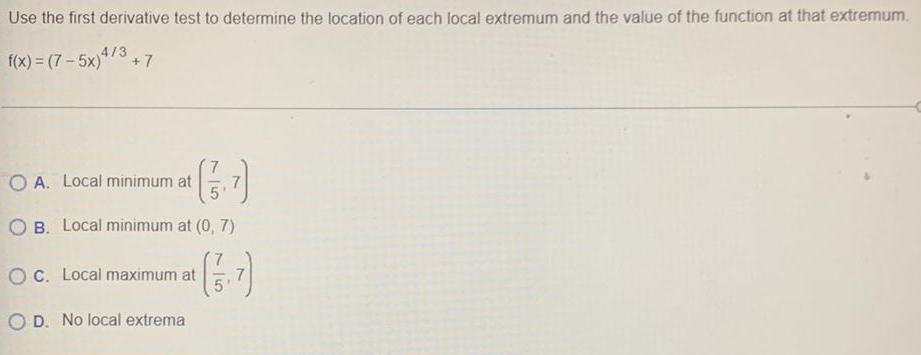Math
Application of derivatives
Use the first derivative test to determine the location of each local extremum and the value of the function at that extremum. f(x) = (7-5x)4/3 +7 A. Local minimum at (7/5,7) B. Local minimum at (0,7) C. Local maximum at (7/5,7) D. No local extrema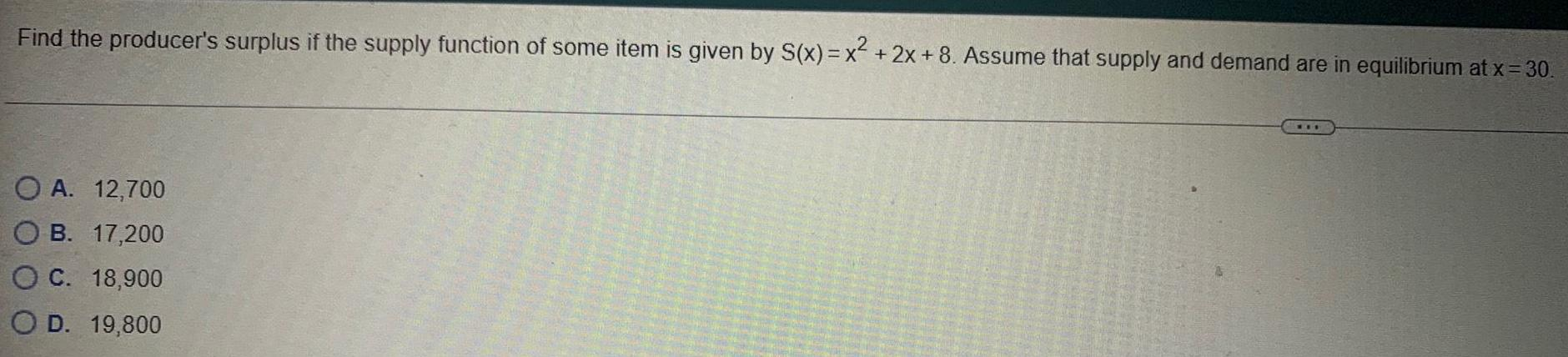Math
Application of derivatives
Find the producer's surplus if the supply function of some item is given by S(x)=x²+2x+8. Assume that supply and demand are in equilibrium at x = 30. A. 12,700 B. 17,200 C. 18,900 D. 19,800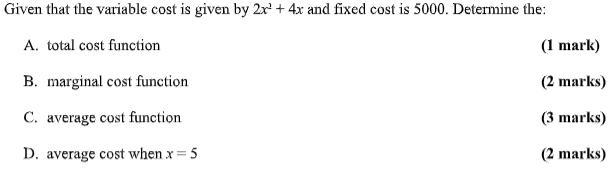Math
Application of derivatives
Given that the variable cost is given by 2x² + 4x and fixed cost is 5000. Determine the: A. total cost function B. marginal cost function C. average cost function D. average cost when x = 5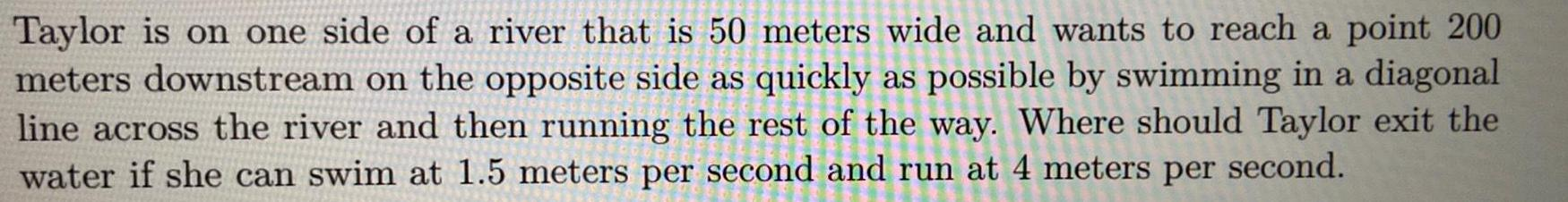Math
Application of derivatives
Taylor is on one side of a river that is 50 meters wide and wants to reach a point 200 meters downstream on the opposite side as quickly as possible by swimming in a diagonal line across the river and then running the rest of the way. Where should Taylor exit the water if she can swim at 1.5 meters per second and run at 4 meters per second.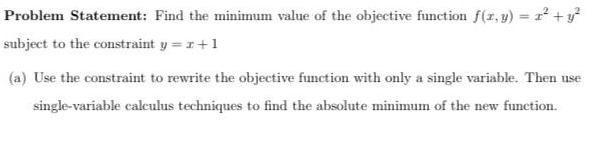Math
Application of derivatives
Find the minimum value of the objective function f(x, y) = x² + y² subject to the constraint y = r +1 (a) Use the constraint to rewrite the objective function with only a single variable. Then use single-variable calculus techniques to find the absolute minimum of the new function.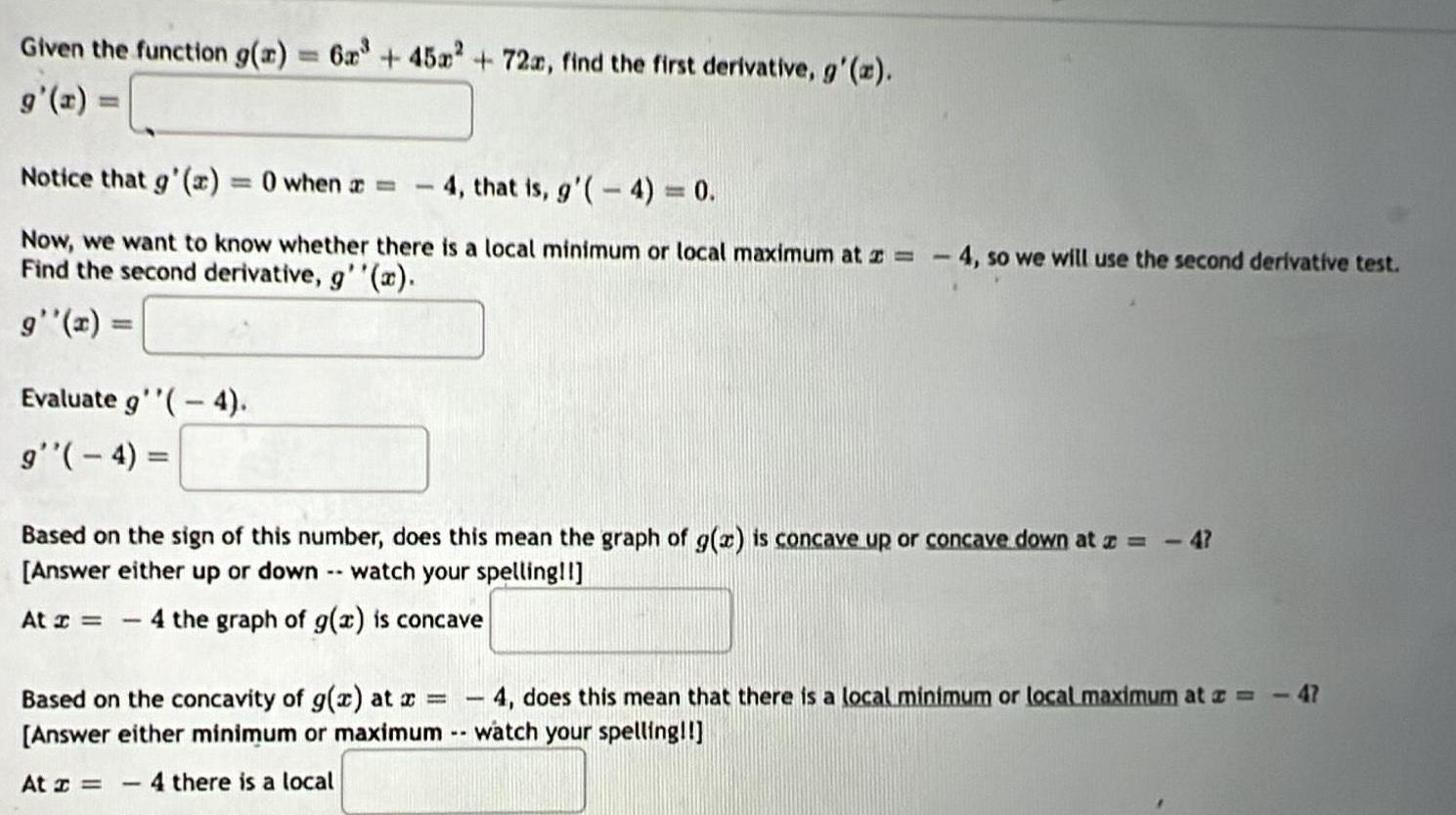Math
Application of derivatives
Given the function g(x) = 6x³ + 45x² + 72x, find the first derivative, g'(x) = 0 when a = 4, that is, g'(-4) = 0. Now, we want to know whether there is a local minimum or local maximum at z = 4, so we will use the second derivative test. Find the second derivative, g''(x). g''(x) = Evaluate g''(-4). g''(-4)= Based on the sign of this number, does this mean the graph of g(x) is concave up or concave down at a = - 4? At = - 4 the graph of g(x) is concave Based on the concavity of g(x) at x = At z = - 4 there is a local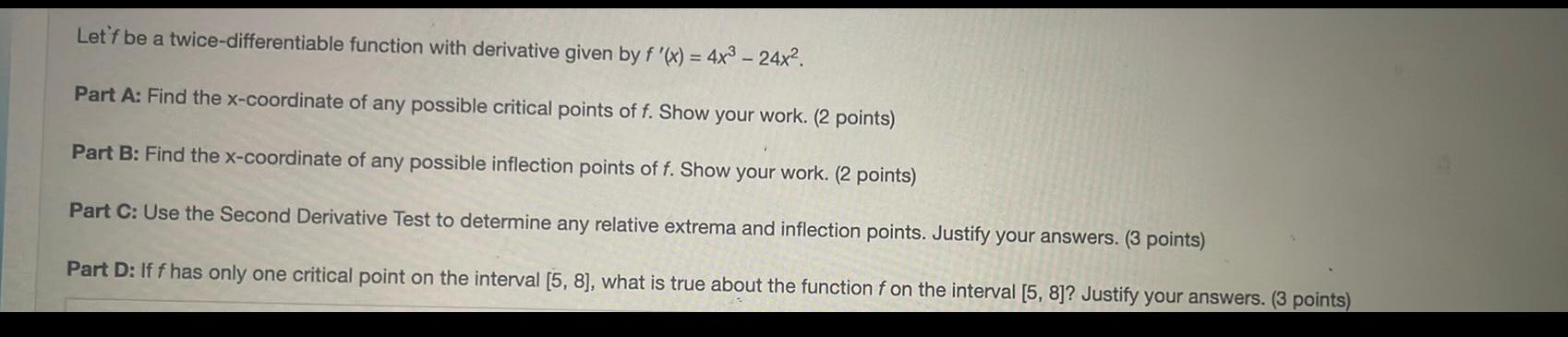Math
Application of derivatives
Let'f be a twice-differentiable function with derivative given by f '(x) = 4x³ - 24x². Part A: Find the x-coordinate of any possible critical points of f. Show your work. Part B: Find the x-coordinate of any possible inflection points of f. Show your work. Part C: Use the Second Derivative Test to determine any relative extrema and inflection points. Justify your answers. Part D: If f has only one critical point on the interval [5, 8], what is true about the function f on the interval [5, 8]? Justify your answers.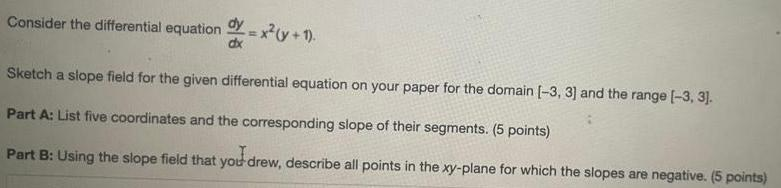Math
Application of derivatives
Consider the differential equation dy/dx = x² (y + 1). Sketch a slope field for the given differential equation on your paper for the domain [-3, 3] and the range [-3, 3]. Part A: List five coordinates and the corresponding slope of their segments. Part B: Using the slope field that you drew, describe all points in the xy-plane for which the slopes are negative.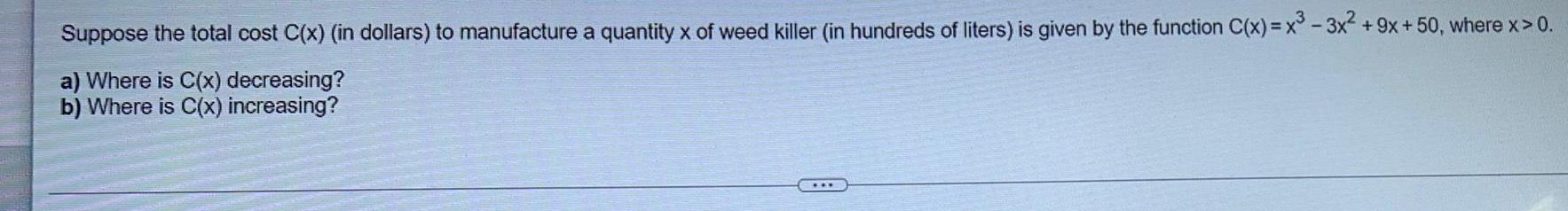Math
Application of derivatives
Suppose the total cost C(x) (in dollars) to manufacture a quantity x of weed killer (in hundreds of liters) is given by the function C(x)= x³ - 3x² +9x +50, where x > 0. a) Where is C(x) decreasing? b) Where is C(x) increasing?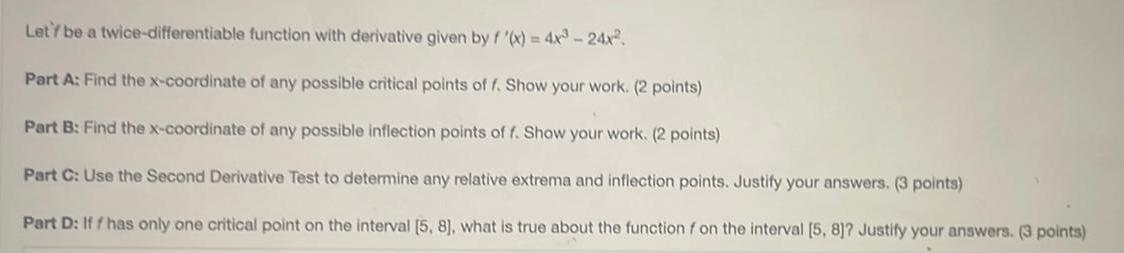Math
Application of derivatives
Let'f be a twice-differentiable function with derivative given by f '(x) = 4x³ - 24x². Part A: Find the x-coordinate of any possible critical points of f. Show your work. Part B: Find the x-coordinate of any possible inflection points of f. Show your work. Part C: Use the Second Derivative Test to determine any relative extrema and inflection points. Justify your answers. Part D: Iff has only one critical point on the interval [5, 8], what is true about the function f on the interval [5, 8]? Justify your answers.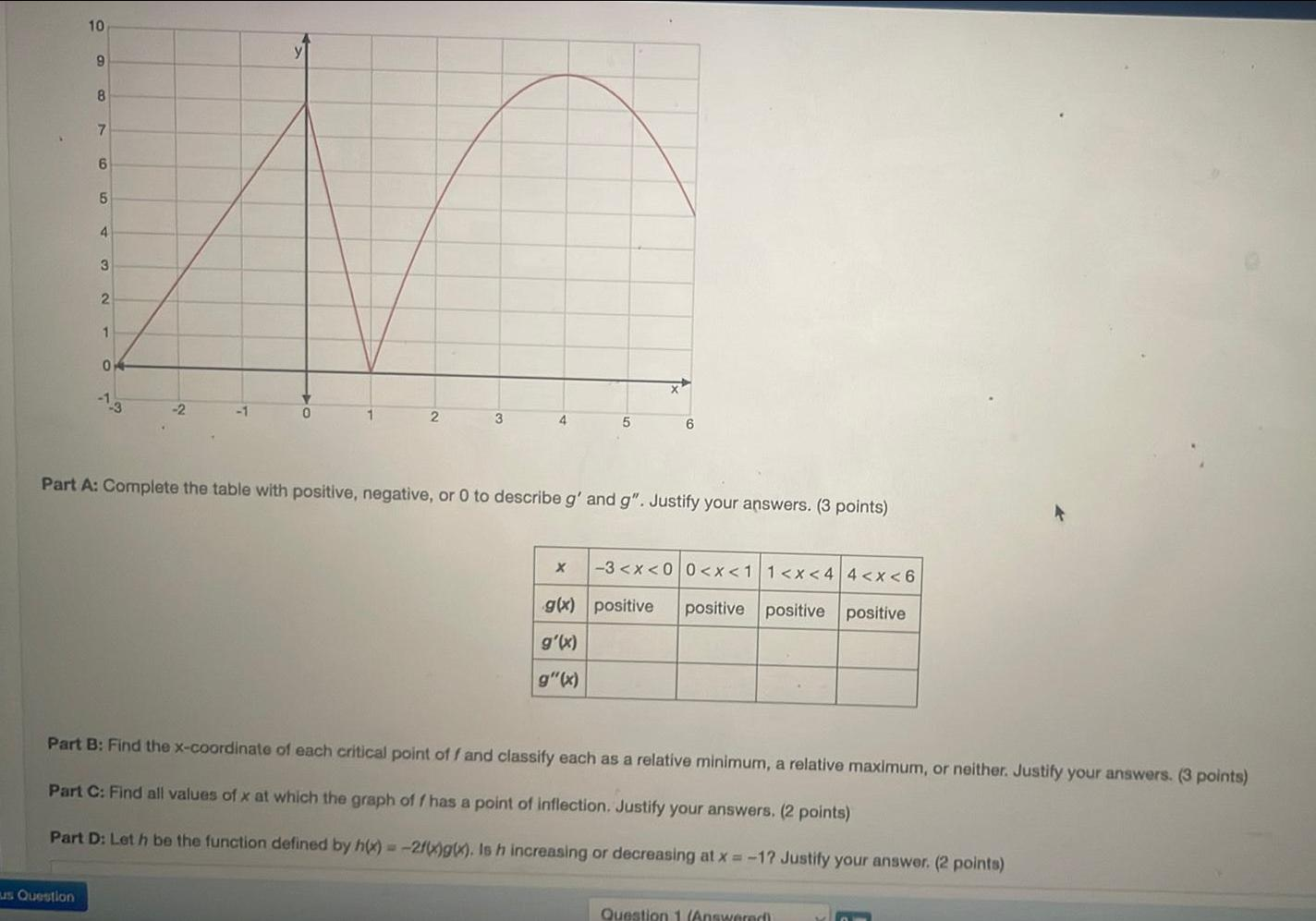Math
Application of derivatives
Complete the table with positive, negative, or 0 to describe g' and g". Justify your answers. positive positive positive positive Part B: Find the x-coordinate of each critical point off and classify each as a relative minimum, a relative maximum, or neither. Justify your answers. Part C: Find all values of x at which the graph of f has a point of inflection. Justify your answers. Part D: Let h be the function defined by h(x) = -2f(x)g(x). Is h increasing or decreasing at x=-1? Justify your answer.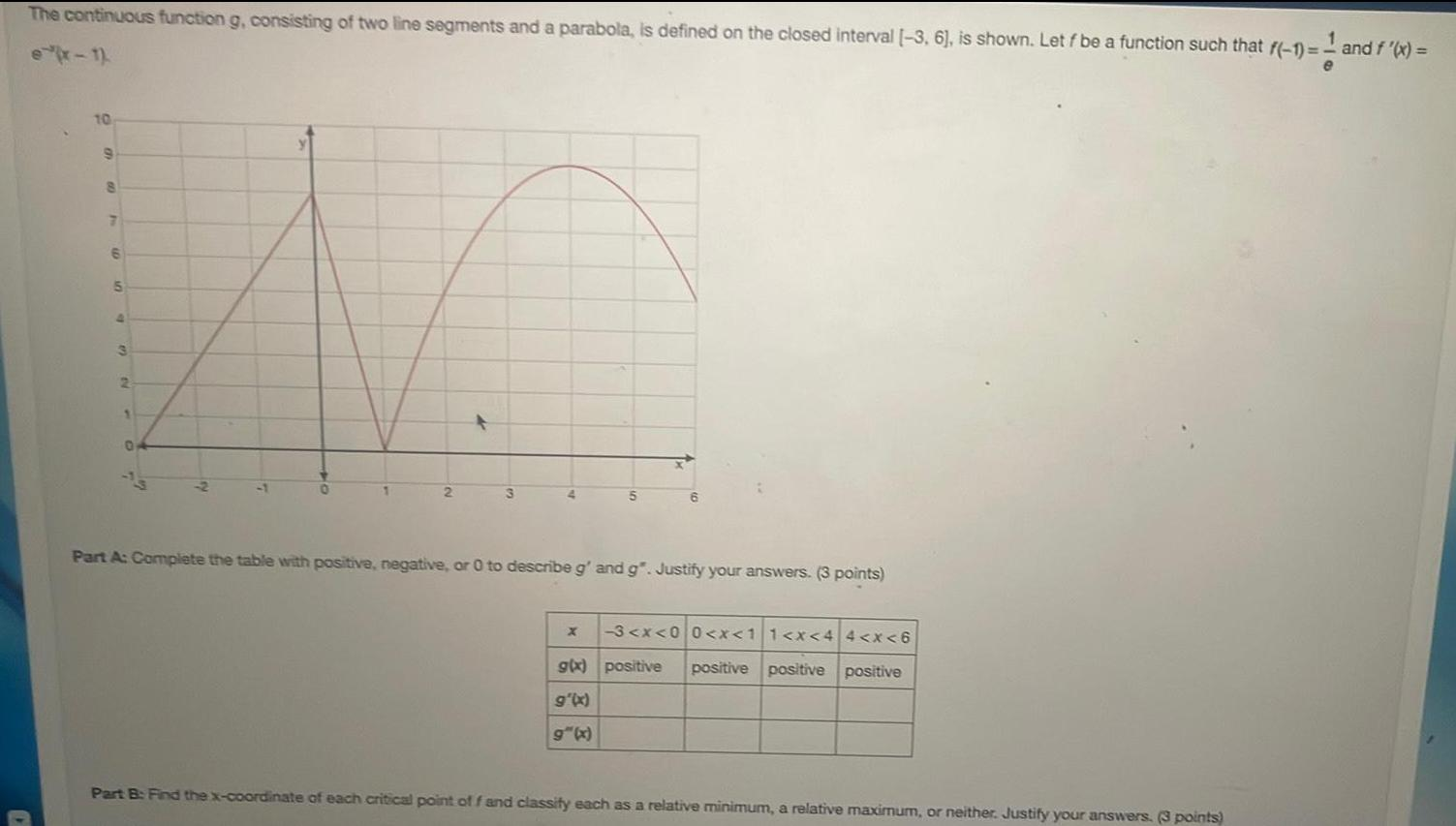Math
Application of derivatives
The continuous function g, consisting of two line segments and a parabola, is defined on the closed interval [-3, 6], is shown. Let f be a function such that f(-1)=- and f '(x) =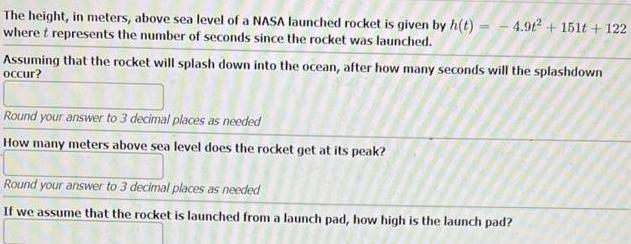Math
Application of derivatives
The height, in meters, above sea level of a NASA launched rocket is given by h(t)=-4.9t² + 151t+122 where t represents the number of seconds since the rocket was launched. Assuming that the rocket will splash down into the ocean, after how many seconds will the splashdown occur? Round your answer to 3 decimal places as needed How many meters above sea level does the rocket get at its peak?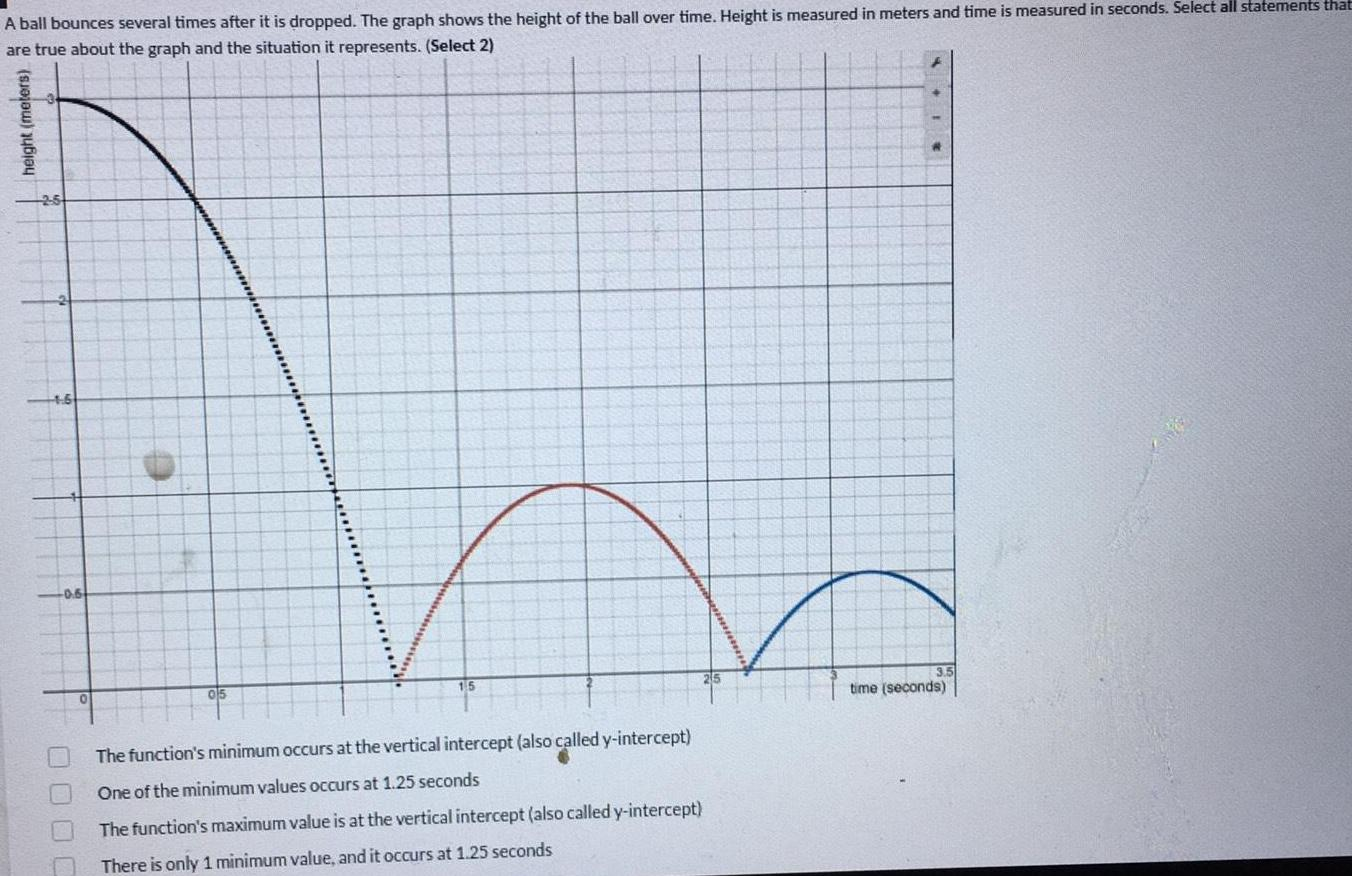Math
Application of derivatives
A ball bounces several times after it is dropped. The graph shows the height of the ball over time. Height is measured in meters and time is measured in seconds. Select all statements that are true about the graph and the situation it represents. The function's minimum occurs at the vertical intercept (also called y-intercept) One of the minimum values occurs at 1.25 seconds The function's maximum value is at the vertical intercept (also called y-intercept) There is only 1 minimum value, and it occurs at 1.25 seconds